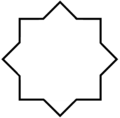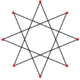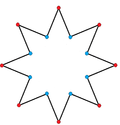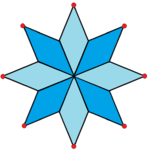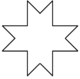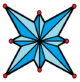# Octagram

Regular octagram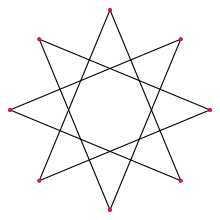A regular octagram
Type Regular star polygon
Edges and vertices 8
Schläfli symbol {8/3}
t{4/3}
Coxeter diagramSymmetry group Dihedral (D8)
Internal angle (degrees) 45°
Dual polygon self
Properties star, cyclic, equilateral, isogonal, isotoxal

In geometry, an octagram is an eight-angled star polygon.

The name octagram combine a Greek numeral prefix, octa-, with the Greek suffix -gram. The -gram suffix derives from γραμμή (grammḗ) meaning "line".

## DetailIn general, an octagram is any self-intersecting octagon (8-sided polygon).

The regular octagram is labeled by the Schläfli symbol {8/3}, which means an 8-sided star, connected by every third point.

## Variations

These variations have a lower dihedral, Dih4, symmetry: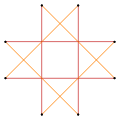Narrow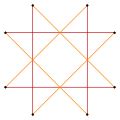Wide(45 degree rotation)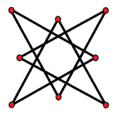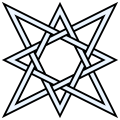Isotoxal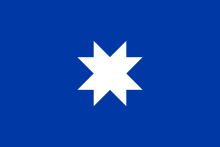An old Flag of Chile contained this octagonal star geometry with edges removed.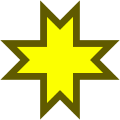The geometry can be adjusted so 3 edges cross at a single point, like the Auseklis symbol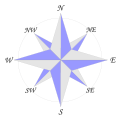An 8-point compass rose can be seen as an octagonal star, with 4 primary points, and 4 secondary points.

The symbol Rub el Hizb is a Unicode glyph ۞  at U+06DE.

## As a quasitruncated square

Deeper truncations of the square can produce isogonal (vertex-transitive) intermediate star polygon forms with equal spaced vertices and two edge lengths. A truncated square is an octagon, t{4}={8}. A quasitruncated square, inverted as {4/3}, is an octagram, t{4/3}={8/3}.

The uniform star polyhedron stellated truncated hexahedron, t'{4,3}=t{4/3,3} has octagram faces constructed from the cube in this way.

Isogonal truncations of square and cube
Regular Quasiregular Isogonal Quasiregular{4}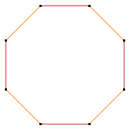t{4}={8}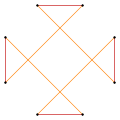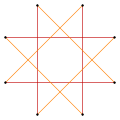t'{4}=t{4/3}={8/3}
Regular Uniform Isogonal Uniform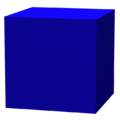{4,3}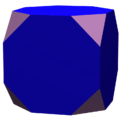t{4,3}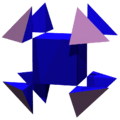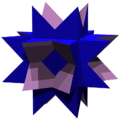t'{4,3}=t{4/3,3}

## Star polygon compounds

There are two regular octagrammic star figures (compounds) of the form {8/k}, the first constructed as two squares {8/2}=2{4}, and second as four degenerate digons, {8/4}=4{2}. There are other isogonal and isotoxal compounds including rectangular and rhombic forms.

Regular Isogonal Isotoxal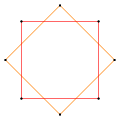a{8}={8/2}=2{4}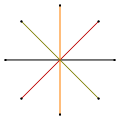{8/4}=4{2}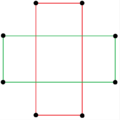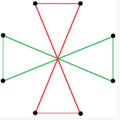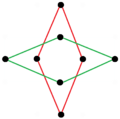## Other presentations of an octagonal star

An octagonal star can be seen as a concave hexadecagon, with internal intersecting geometry erased. It can also be dissected by radial lines.

2{4} {8/3}